This is “Statistical Functions”, section 2.2 from the book Using Microsoft Excel (v. 1.0). For details on it (including licensing), click here.

Has this book helped you? Consider passing it on:
Creative Commons supports free culture from music to education. Their licenses helped make this book available to you.
DonorsChoose.org helps people like you help teachers fund their classroom projects, from art supplies to books to calculators.

## 2.2 Statistical Functions

### Learning Objectives

1. Use the SUM function to calculate totals.
2. Use absolute references to calculate percent of totals.
3. Use the COUNT function to count cell locations with numerical values.
4. Use the AVERAGE function to calculate the arithmetic mean.
5. Use the MAX and MIN functions to find the highest and lowest values in a range of cells.
6. Learn how to copy and paste formulas without formats applied to a cell location.
7. Learn how to set a multiple level sort sequence for data sets that have duplicate values or outputs.

In addition to formulas, another way to conduct mathematical computations in Excel is through functions. Statistical functions apply a mathematical process to a group of cells in a worksheet. For example, the SUM function is used to add the values contained in a range of cells. A list of commonly used statistical functions is shown in Table 2.4 "Commonly Used Statistical Functions". Functions are more efficient than formulas when you are applying a mathematical process to a group of cells. If you use a formula to add the values in a range of cells, you would have to add each cell location to the formula one at a time. This can be very time-consuming if you have to add the values in a few hundred cell locations. However, when you use a function, you can highlight all the cells that contain values you wish to sum in just one step. This section demonstrates a variety of statistical functions that we will add to the Personal Budget workbook. In addition to demonstrating functions, this section also reviews percent of total calculations and the use of absolute references.

Table 2.4 Commonly Used Statistical Functions

Function Output
ABS The absolute value of a number
AVERAGE The average or arithmetic mean for a group of numbers
COUNT The number of cell locations in a range that contain a numeric character
COUNTA The number of cell locations in a range that contain a text or numeric character
MAX The highest numeric value in a group of numbers
MEDIAN The middle number in a group of numbers (half the numbers in the group are higher than the median and half the numbers in the group are lower than the median)
MIN The lowest numeric value in a group of numbers
MODE The number that appears most frequently in a group of numbers
PRODUCT The result of multiplying all the values in a range of cell locations
SQRT The positive square root of a number
STDEV.S The standard deviation for a group of numbers based on a sample
SUM The total of all numeric values in a group

## The SUM Function

Follow-along file: Continue with Excel Objective 2.00. (Use file Excel Objective 2.03 if starting here.)

The SUM function is used when you need to calculate totals for a range of cells or a group of selected cells on a worksheet. With regard to the Budget Detail worksheet, we will use the SUM function to calculate the totals in row 12. It is important to note that there are several methods for adding a function to a worksheet, which will be demonstrated throughout the remainder of this chapter. The following illustrates how a function can be added to a worksheet by typing it into a cell location:

1. Click the Budget Detail worksheet tab to open the worksheet.
2. Click cell C12.
3. Type an equal sign (=).
4. Type the function name SUM.
5. Type an open parenthesis (().
6. Click cell C3 and drag down to cell C11. This places the range C3:C11 into the function.
7. Type a closing parenthesis ()).
8. Press the ENTER key. The function calculates the total for the Monthly Spend column, which is \$1,496.

Figure 2.11 "Adding the SUM Function to the Budget Detail Worksheet" shows the appearance of the SUM function added to the Budget Detail worksheet before pressing the ENTER key.

Figure 2.11 Adding the SUM Function to the Budget Detail Worksheet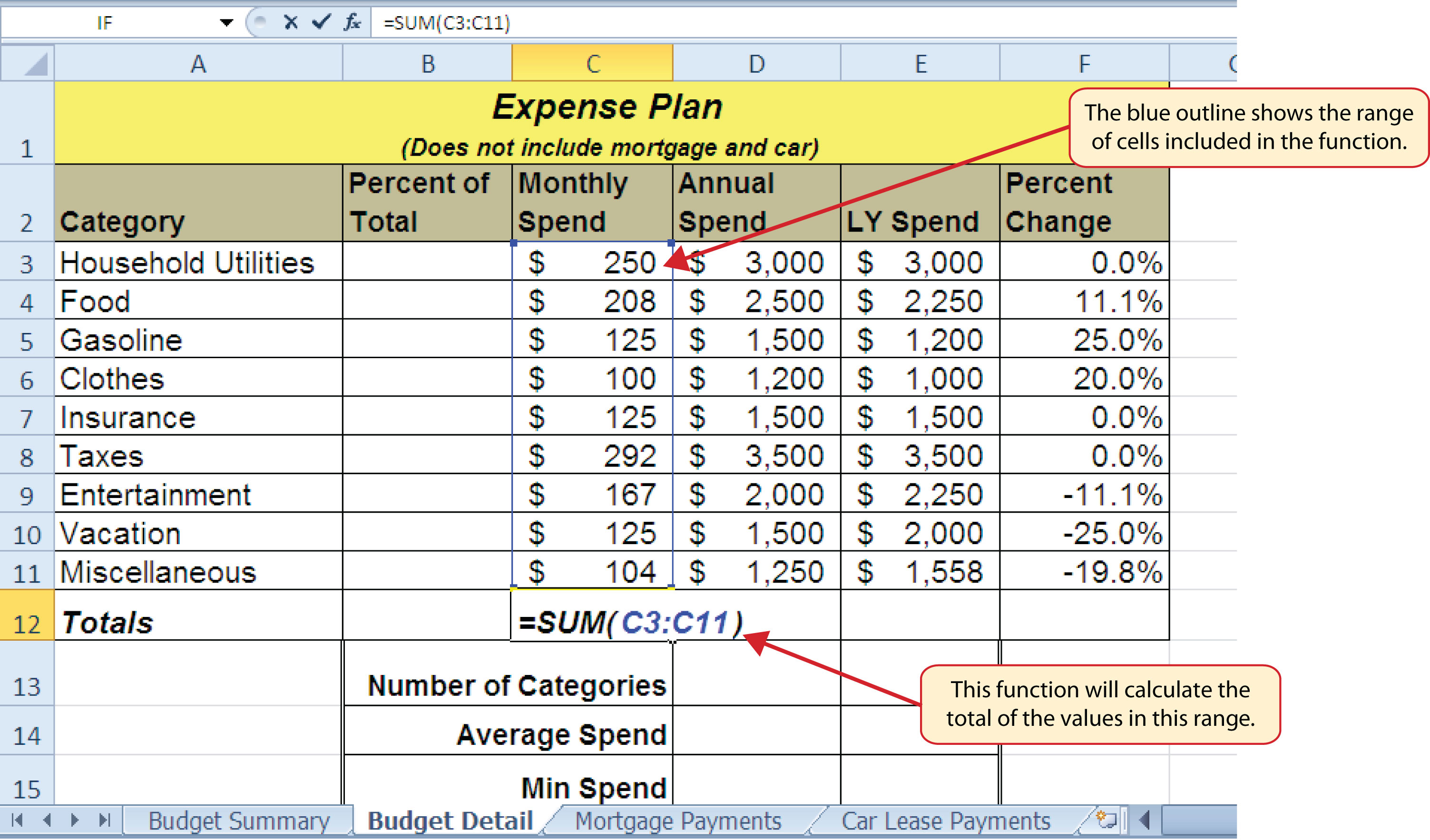As shown in Figure 2.11 "Adding the SUM Function to the Budget Detail Worksheet", the SUM function was added to cell C12. However, this function is also needed to calculate the totals in the Annual Spend and LY Spend columns. The function can be copied and pasted into these cell locations because of relative referencing. Relative referencing serves the same purpose for functions as it does for formulas. The following demonstrates how the total row is completed:

1. Click cell C12 in the Budget Detail worksheet.
2. Click the Copy button in the Home tab of the Ribbon.
3. Highlight cells D12 and E12.
4. Click the Paste button in the Home tab of the Ribbon. This pastes the SUM function into cells D12 and E12 and calculates the totals for these columns.
5. Click cell F11.
6. Click the Copy button in the Home tab of the Ribbon.
7. Click cell F12, then click the Paste button in the Home tab of the Ribbon. Since we now have totals in row 12, we can paste the percent change formula into this row.

Figure 2.12 "Results of the SUM Function in the Budget Detail Worksheet" shows the output of the SUM function that was added to cells C12, D12, and E12. In addition, the percent change formula was copied and pasted into cell F12. Notice that this version of the budget is planning a 1.7% decrease in spending compared to last year.

Figure 2.12 Results of the SUM Function in the Budget Detail Worksheet### Integrity Check

Cell Ranges in Statistical Functions

When you intend to use a statistical function on a range of cells in a worksheet, make sure there are two cell locations separated by a colon and not a comma. If you enter two cell locations separated by a comma, the function will produce an output but it will be applied to only two cell locations instead of a range of cells. For example, the SUM function shown in Figure 2.13 "SUM Function Adding Two Cell Locations" will add only the values in cells C3 and C11, not the range C3:C11.

Figure 2.13 SUM Function Adding Two Cell Locations## Absolute References (Calculating Percent of Totals)

Follow-along file: Continue with Excel Objective 2.00. (Use file Excel Objective 2.04 if starting here.)

Since totals were added to row 12 of the Budget Detail worksheet, a percent of total calculation can be added to Column B beginning in cell B3. The percent of total calculation shows the percentage for each value in the Annual Spend column with respect to the total in cell D12. However, after the formula is created, it will be necessary to turn off Excel’s relative referencing feature before copying and pasting the formula to the rest of the cell locations in the column. Turning off Excel’s relative referencing feature is accomplished through an absolute reference. The following steps explain how this is done:

1. Click cell B3 in the Budget Detail worksheet.
2. Type an equal sign (=).
3. Click cell D3.
4. Type a forward slash (/).
5. Click cell D12.
6. Press the ENTER key. You will see that Household Utilities represents 16.7% of the Annual Spend budget (see Figure 2.14 "Adding a Formula to Calculate the Percent of Total").

Figure 2.14 Adding a Formula to Calculate the Percent of Total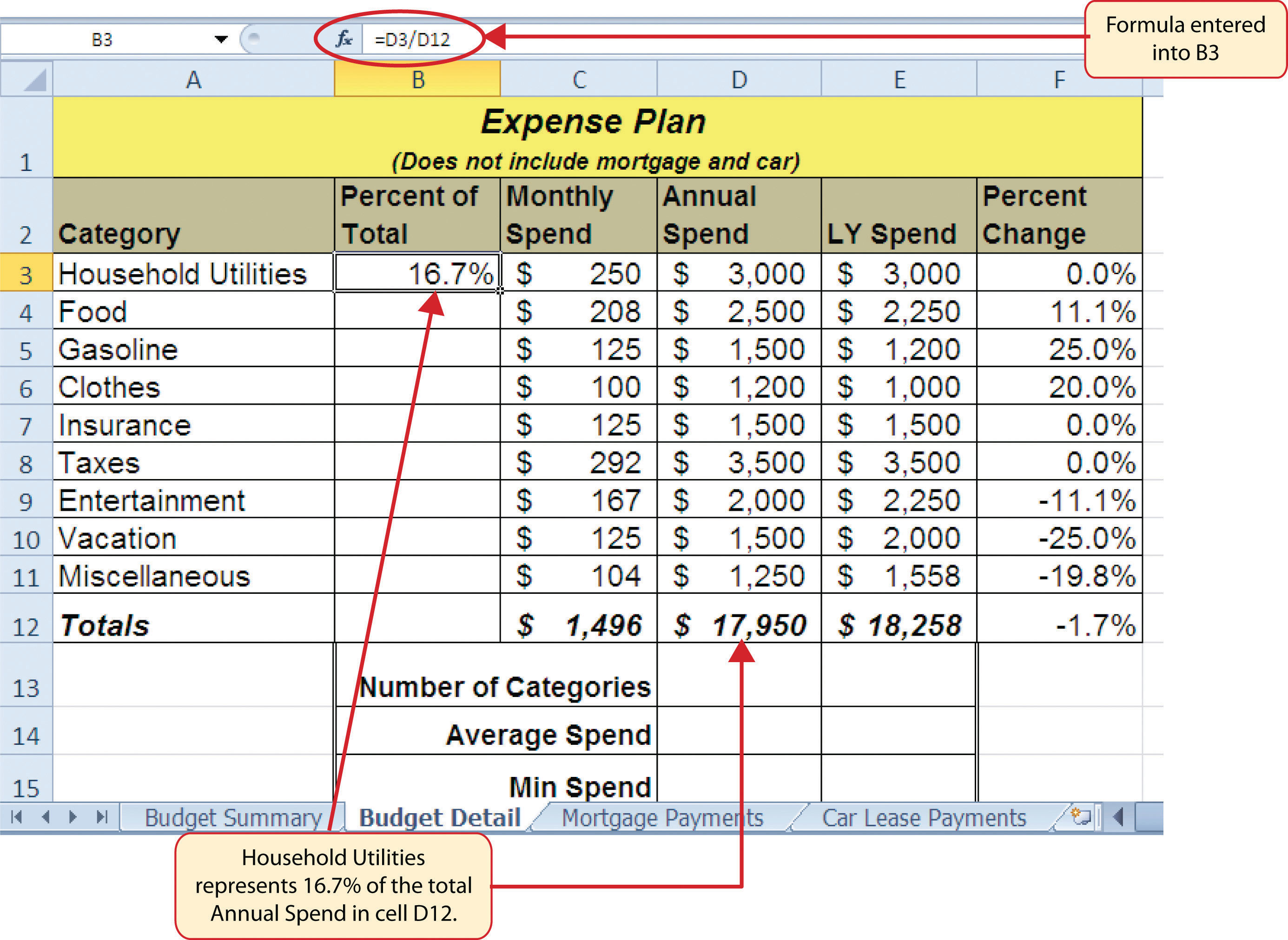Figure 2.14 "Adding a Formula to Calculate the Percent of Total" shows the completed formula that is calculating the percentage that Household Utilities Annual Spend represents to the total Annual Spend for the budget (see cell B3). Normally, we would copy this formula and paste it into the range B4:B11. However, because of relative referencing, both cell references will increase by one row as the formula is pasted into the cells below B3. This is fine for the first cell reference in the formula (D3) but not for the second cell reference (D12). Figure 2.15 "#DIV/0 Error from Relative Referencing" illustrates what happens if we paste the formula into the range B4:B12 in its current state. Notice that Excel produces the #DIV/0Error code displayed in a cell containing a formula or function that is trying to divide by zero. error code. This means that Excel is trying to divide a number by zero, which is impossible. Looking at the formula in cell B4, you see that the first cell reference was changed from D3 to D4. This is fine because we now want to divide the Annual Spend for Insurance by the total Annual Spend in cell D12. However, Excel has also changed the D12 cell reference to D13. Because cell location D13 is blank, the formula produces the #DIV/0 error code.

Figure 2.15 #DIV/0 Error from Relative ReferencingTo eliminate the divide-by-zero error shown in Figure 2.15 "#DIV/0 Error from Relative Referencing", we must add an absolute reference to cell D12 in the formula. An absolute referencePrevents Excel from using relative referencing to change a cell reference used in a formula or function; an absolute reference is applied to a cell reference by typing a dollar sign (\$) in front of the column letter and row number. prevents relative referencing from changing a cell reference in a formula. This is also referred to as locking a cell. The following explains how this is accomplished:

1. Double click cell B3.
2. Place the mouse pointer in front of D12 and click. The blinking cursor should be in front of the D in the cell reference D12.
3. Press the F4 key. You will see a dollar sign (\$) added in front of the column letter D and the row number 12. You can also type the dollar signs in front of the column letter and row number.
4. Press the ENTER key.
5. Click cell B3.
6. Click the Copy button in the Home tab of the Ribbon.
7. Highlight the range B4:B11.
8. Click the Paste button in the Home tab of the Ribbon.

Figure 2.16 "Adding an Absolute Reference to a Cell Reference in a Formula" shows the percent of total formula with an absolute reference added to D12. Notice that in cell B4, the cell reference remains D12 instead of changing to D13 as shown in Figure 2.15 "#DIV/0 Error from Relative Referencing". Also, you will see that the percentages are being calculated in the rest of the cells in the column, and the divide-by-zero error is now eliminated.

Figure 2.16 Adding an Absolute Reference to a Cell Reference in a Formula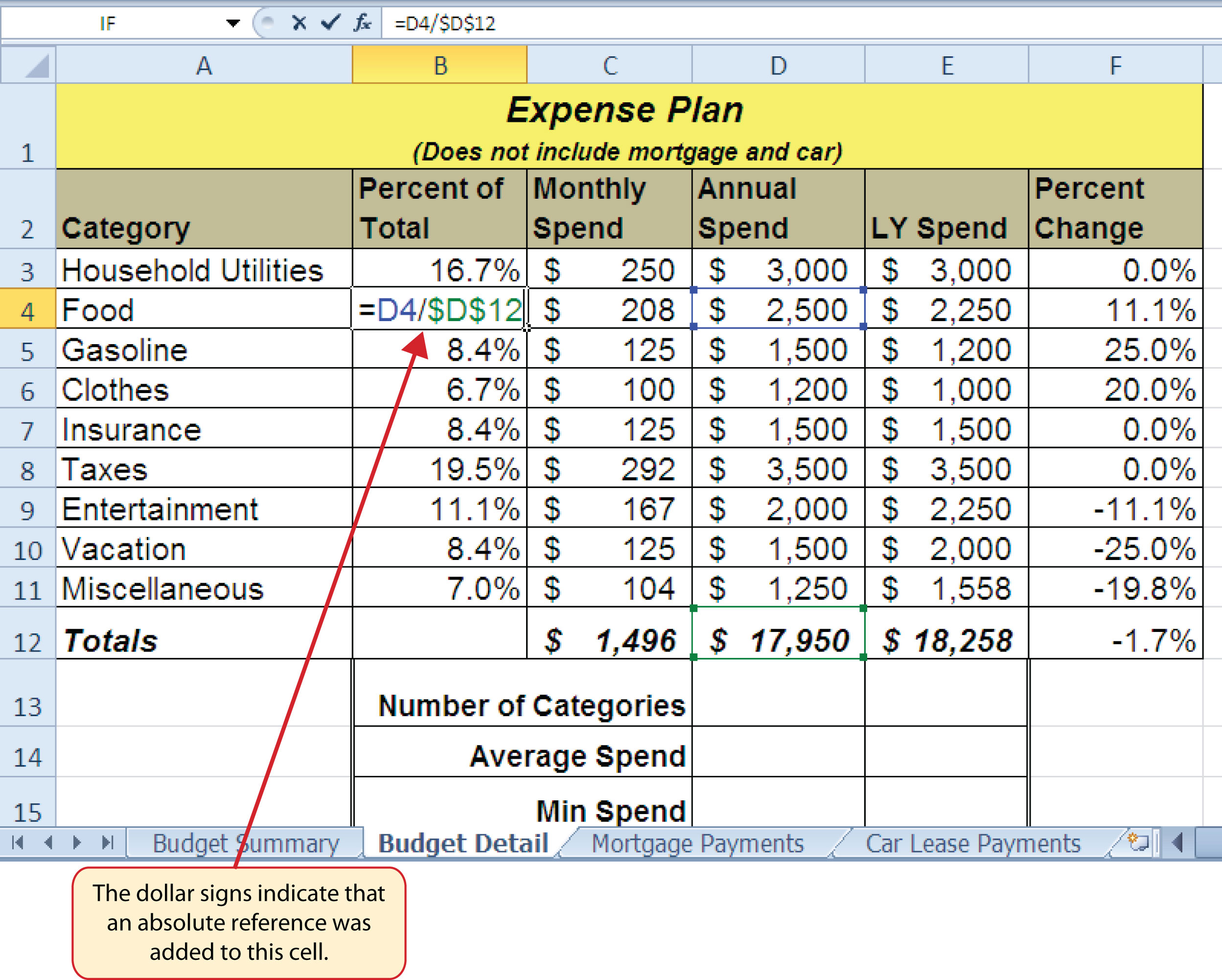### Skill Refresher: Absolute References

1. Click in front of the column letter of a cell reference in a formula or function that you do not want altered when the formula or function is pasted into a new cell location.
2. Press the F4 key or type a dollar sign (\$) in front of the column letter and row number of the cell reference.

## The COUNT Function

Follow-along file: Continue with Excel Objective 2.00. (Use file Excel Objective 2.05 if starting here.)

The next function that we will add to the Budget Detail worksheet is the COUNT function. The COUNT function is used to determine how many cells in a range contain a numeric entry. For the Budget Detail worksheet, we will use the COUNT function to count the number of items that are planned in the Annual Spend column (Column D). The following explains how the COUNT function is added to the worksheet by using the function list:

1. Click cell D13 in the Budget Detail worksheet.
2. Type an equal sign (=).
3. Type the letter C.
4. Click the down arrow on the scroll bar of the function list (see Figure 2.17 "Using the Function List to Add the COUNT Function") and find the word COUNT.
5. Double click the word COUNT from the function list.
6. Highlight the range D3:D11.
7. Type a closing parenthesis ()).
8. Press the ENTER key. The function produces an output of 9 since there are 9 items planned on the worksheet.

Figure 2.17 "Using the Function List to Add the COUNT Function" shows the function list box that appears after completing steps 2 and 3 for the COUNT function. The function list provides an alternative method for adding a function to a worksheet.

Figure 2.17 Using the Function List to Add the COUNT Function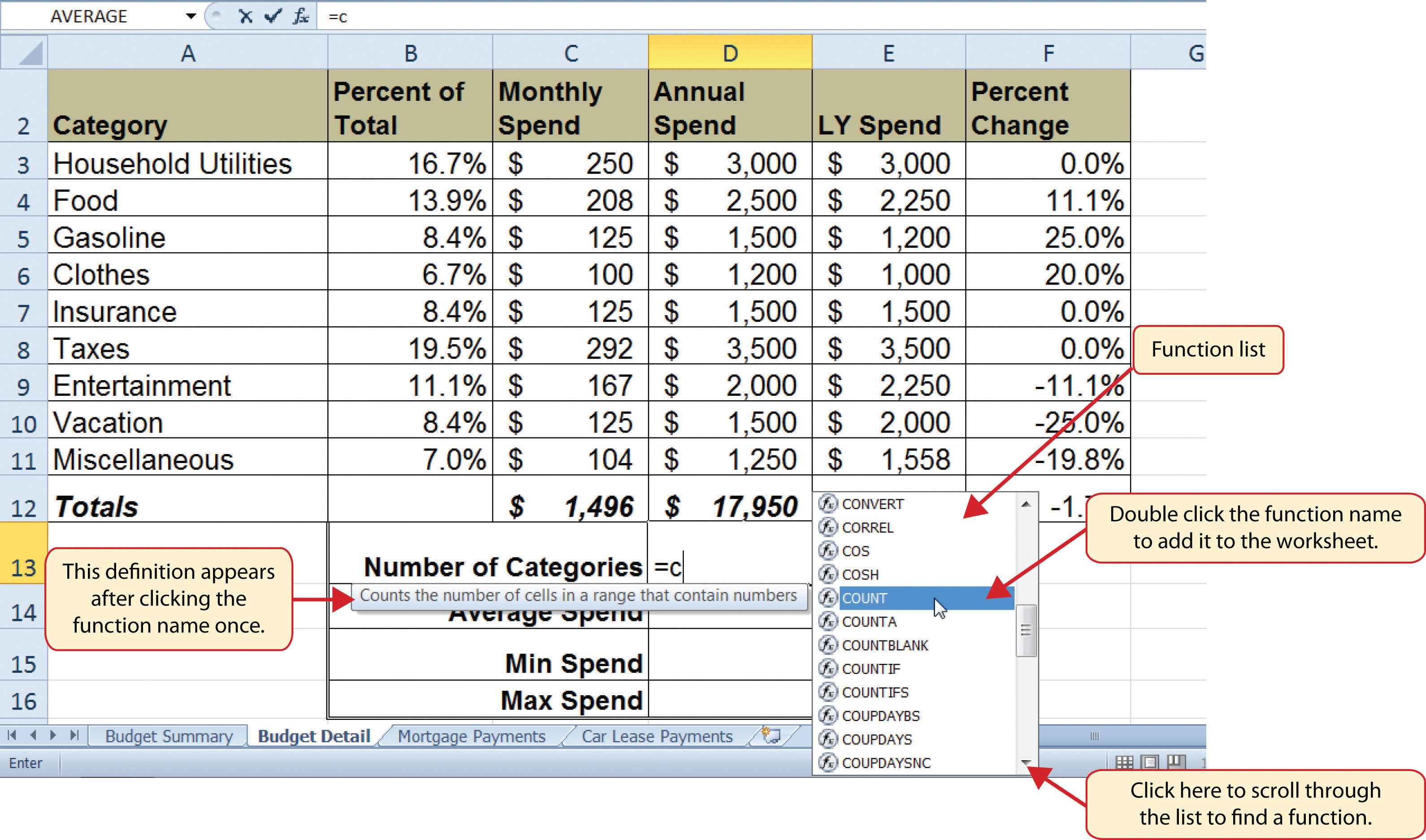Figure 2.18 "Completed COUNT Function in the Budget Detail Worksheet" shows the output of the COUNT function after pressing the ENTER key. The function counts the number of cells in the range D3:D11 that contain a numeric value. The result of 9 indicates that there are 9 categories planned for this budget.

Figure 2.18 Completed COUNT Function in the Budget Detail Worksheet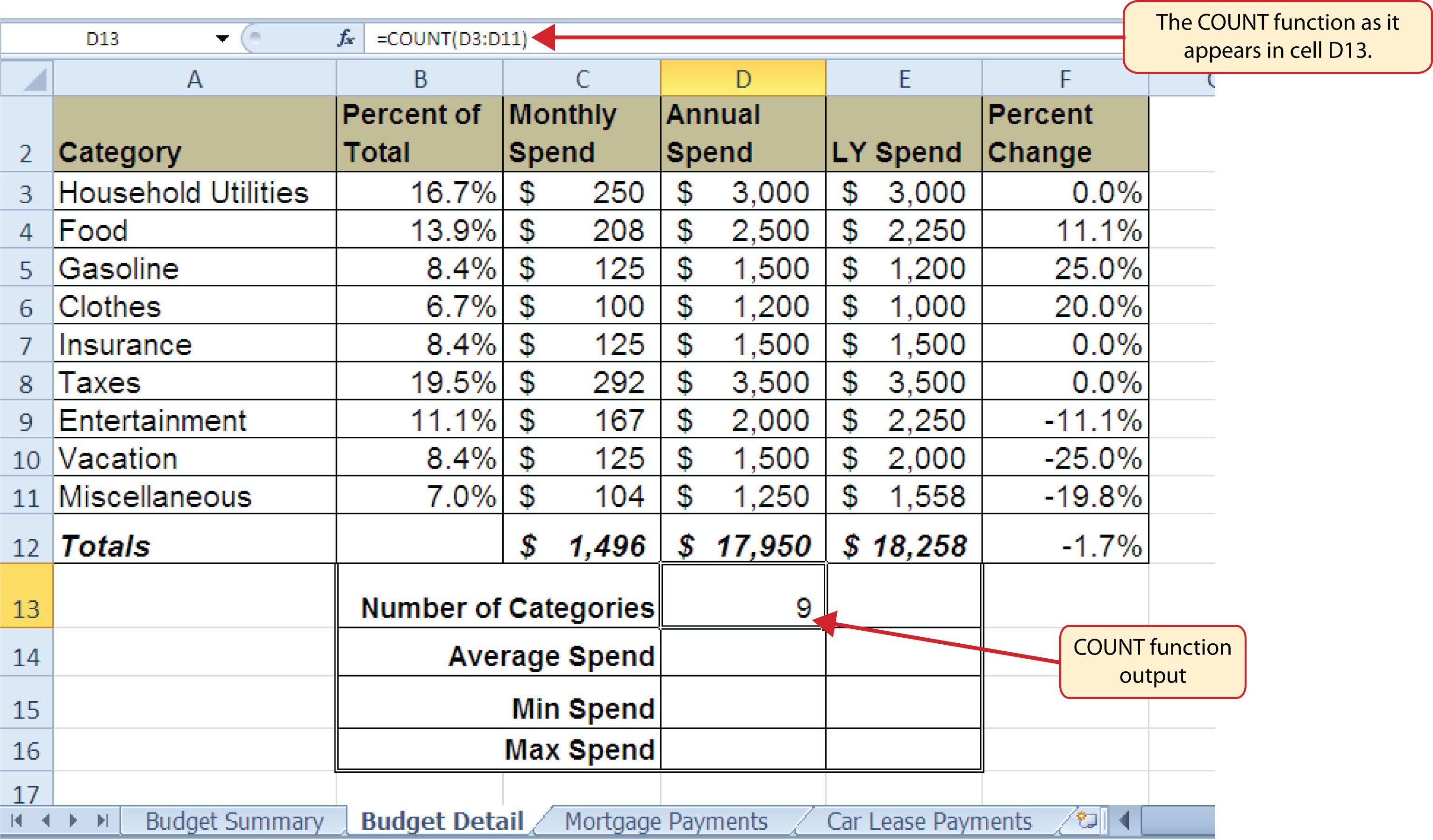## The AVERAGE Function

Follow-along file: Continue with Excel Objective 2.00. (Use file Excel Objective 2.06 if starting here.)

The next function we will add to the Budget Detail worksheet is the AVERAGE function. This function is used to calculate the arithmetic mean for a group of numbers. For the Budget Detail worksheet, we will use the function to calculate the average of the values in the Annual Spend column. We will add this to the worksheet by using the Function Library. The following steps explain how this is accomplished:

1. Click cell D14 in the Budget Detail worksheet.
2. Click the Formulas tab on the Ribbon.
3. Click the More Functions button in the Function Library group of commands.
4. Place the mouse pointer over the Statistical option from the drop-down list of options.
5. Click the AVERAGE function name from the list of functions that appear in the menu (see Figure 2.19 "Selecting the AVERAGE Function from the Function Library"). This opens the Function Arguments dialog box.
6. Click the Collapse Dialog button in the Function Arguments dialog box (see Figure 2.20 "Function Arguments Dialog Box").
7. Highlight the range D3:D11.
8. Click the Expand Dialog button in the Function Arguments dialog box (see Figure 2.21 "Selecting a Range from the Function Arguments Dialog Box"). You can also press the ENTER key to get the same result.
9. Click the OK button on the Function Arguments dialog box. This adds the AVERAGE function to the worksheet.

Figure 2.19 "Selecting the AVERAGE Function from the Function Library" illustrates how a function is selected from the Function Library in the Formulas tab of the Ribbon.

Figure 2.19 Selecting the AVERAGE Function from the Function Library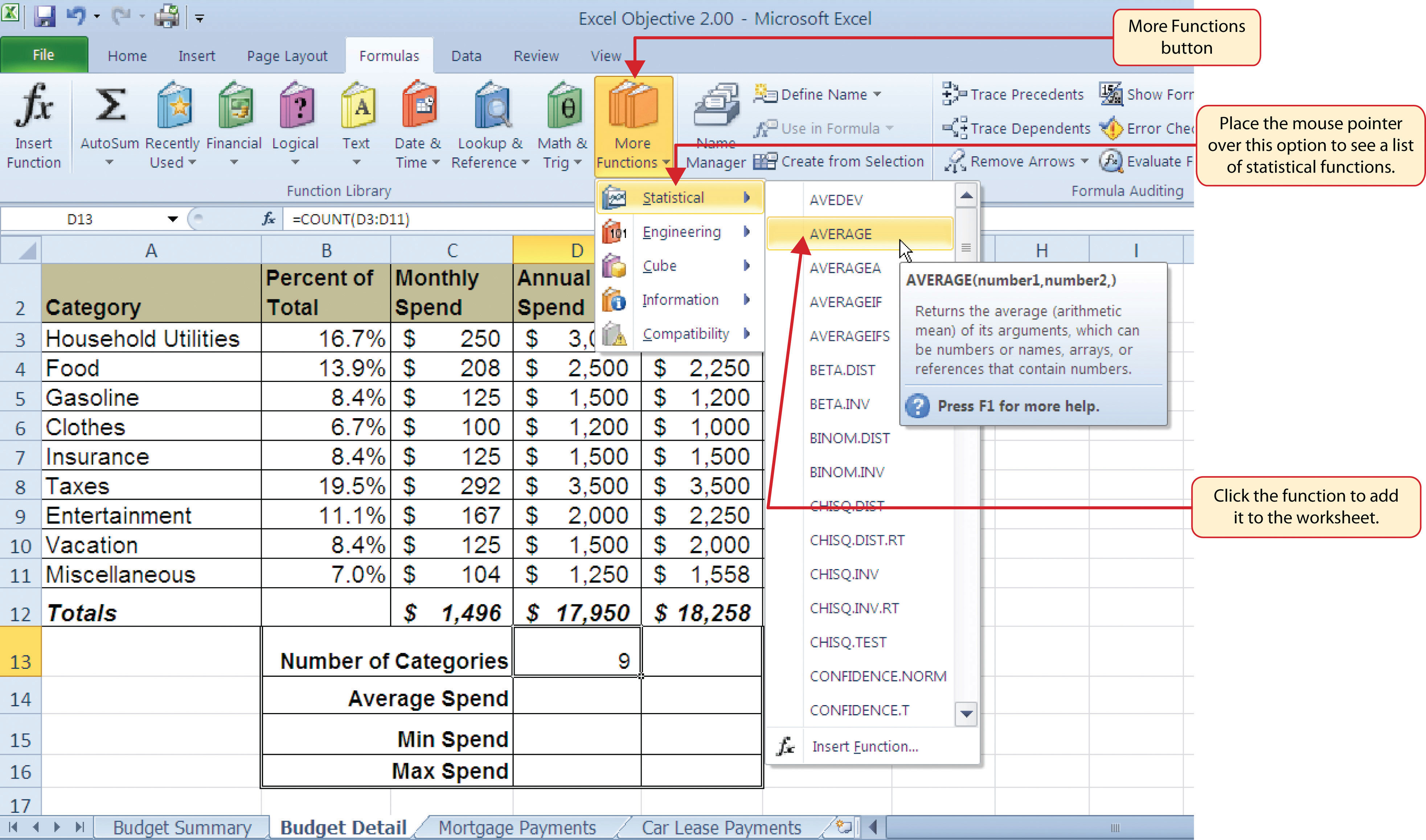Figure 2.20 "Function Arguments Dialog Box" shows the Function Arguments dialog box. This appears after a function is selected from the Function Library. The Collapse Dialog button is used to hide the dialog box so a range of cells can be highlighted on the worksheet and then added to the function.

Figure 2.20 Function Arguments Dialog Box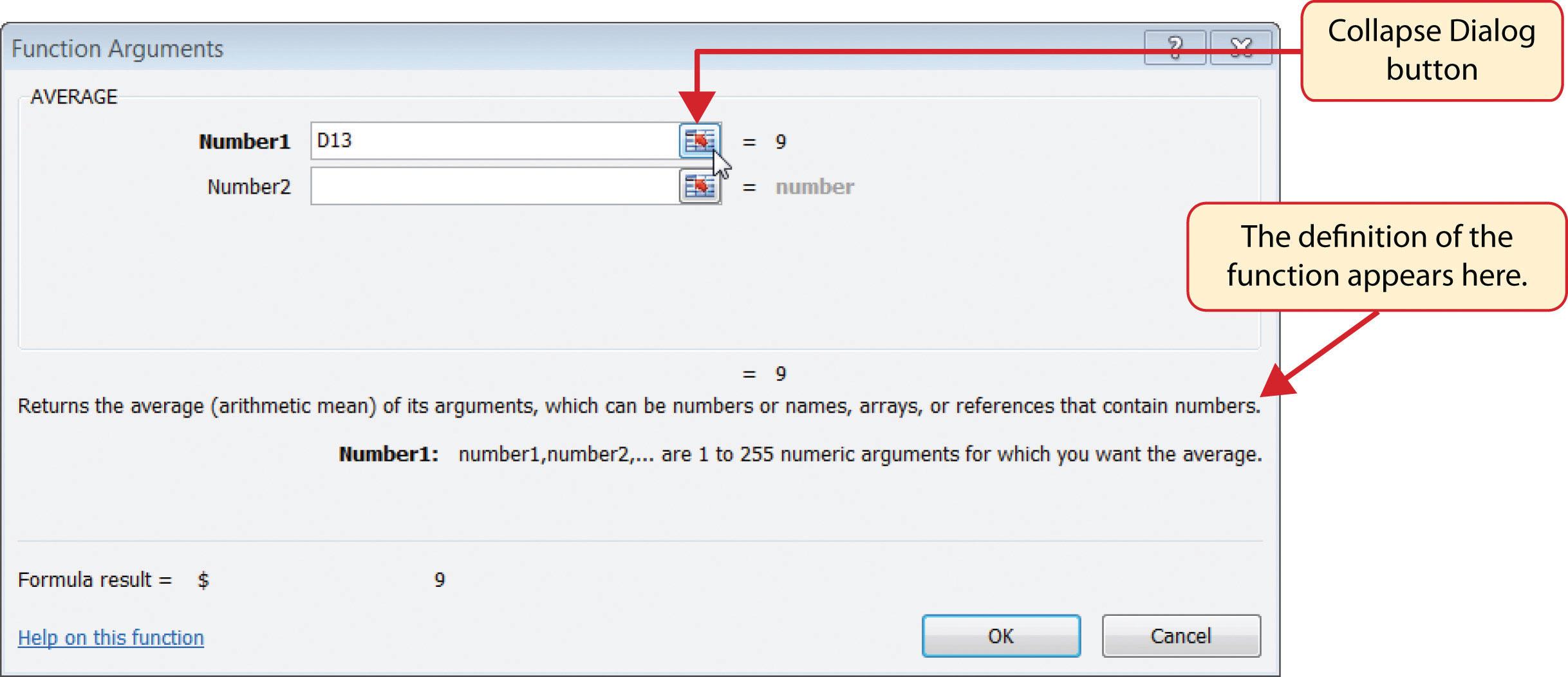Figure 2.21 "Selecting a Range from the Function Arguments Dialog Box" shows how a range of cells can be selected from the Function Arguments dialog box once it has been collapsed.

Figure 2.21 Selecting a Range from the Function Arguments Dialog Box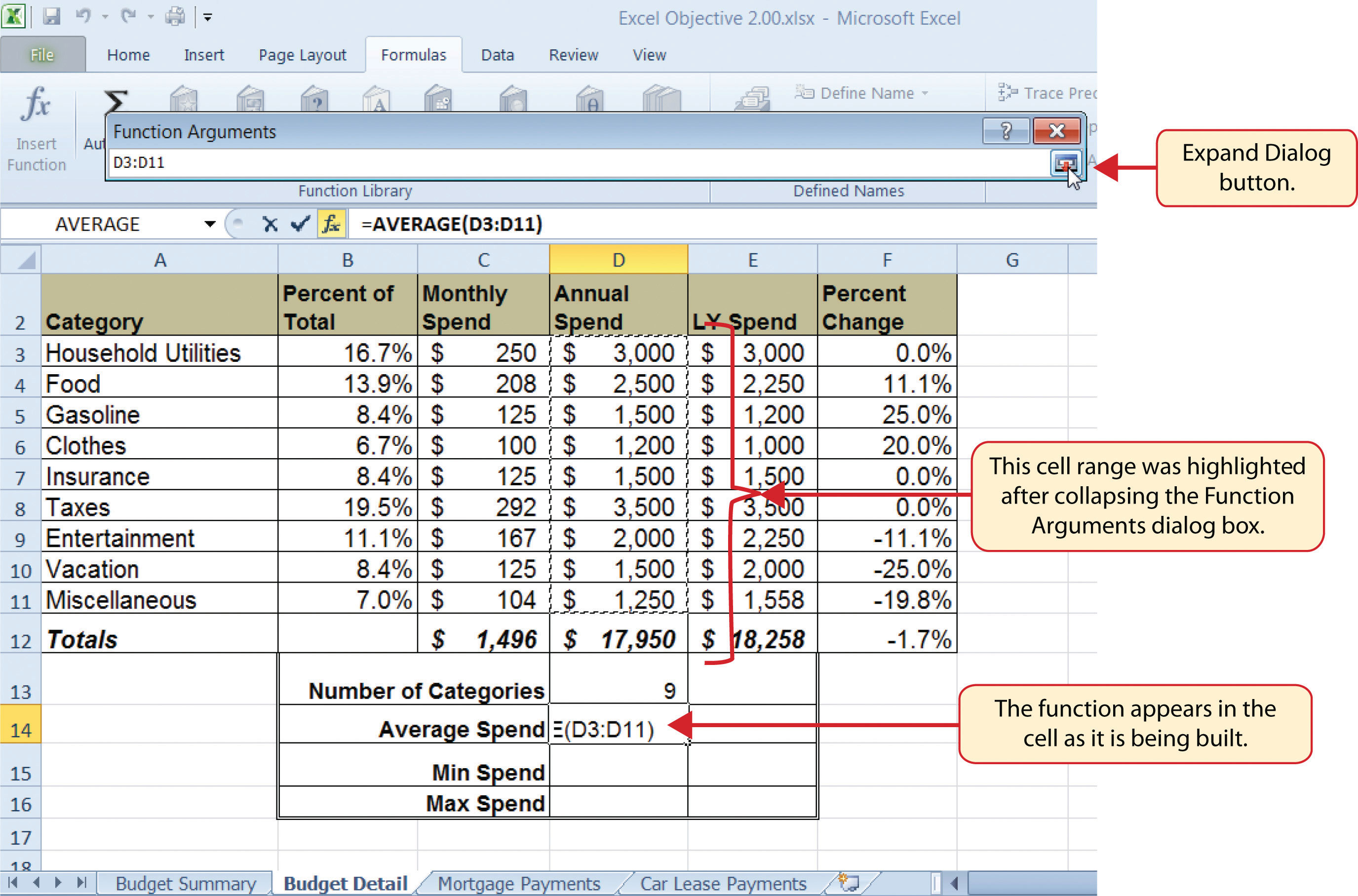Figure 2.22 "Function Arguments Dialog Box after a Cell Range Is Defined for a Function" shows the Function Arguments dialog box after the cell range is defined for the AVERAGE function. The dialog box shows the result of the function before it is added to the cell location. This allows you to assess the function output to determine whether it makes sense before adding it to the worksheet.

Figure 2.22 Function Arguments Dialog Box after a Cell Range Is Defined for a Function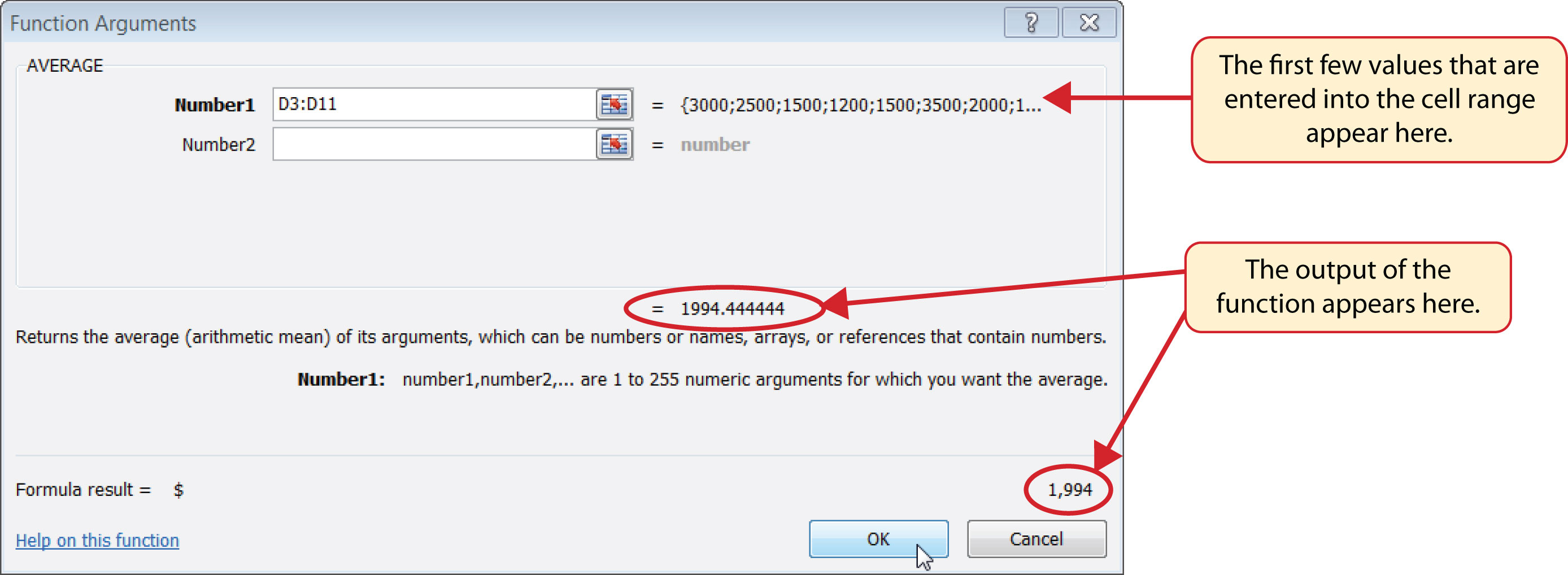Figure 2.23 "Completed AVERAGE Function" shows the completed AVERAGE function in the Budget Detail worksheet. The output of the function shows that on average we expect to spend \$1,994 for each of the categories listed in Column A of the budget. This average spend calculation per category can be used as an indicator to determine which categories are costing more or less than the average budgeted spend dollars.

Figure 2.23 Completed AVERAGE Function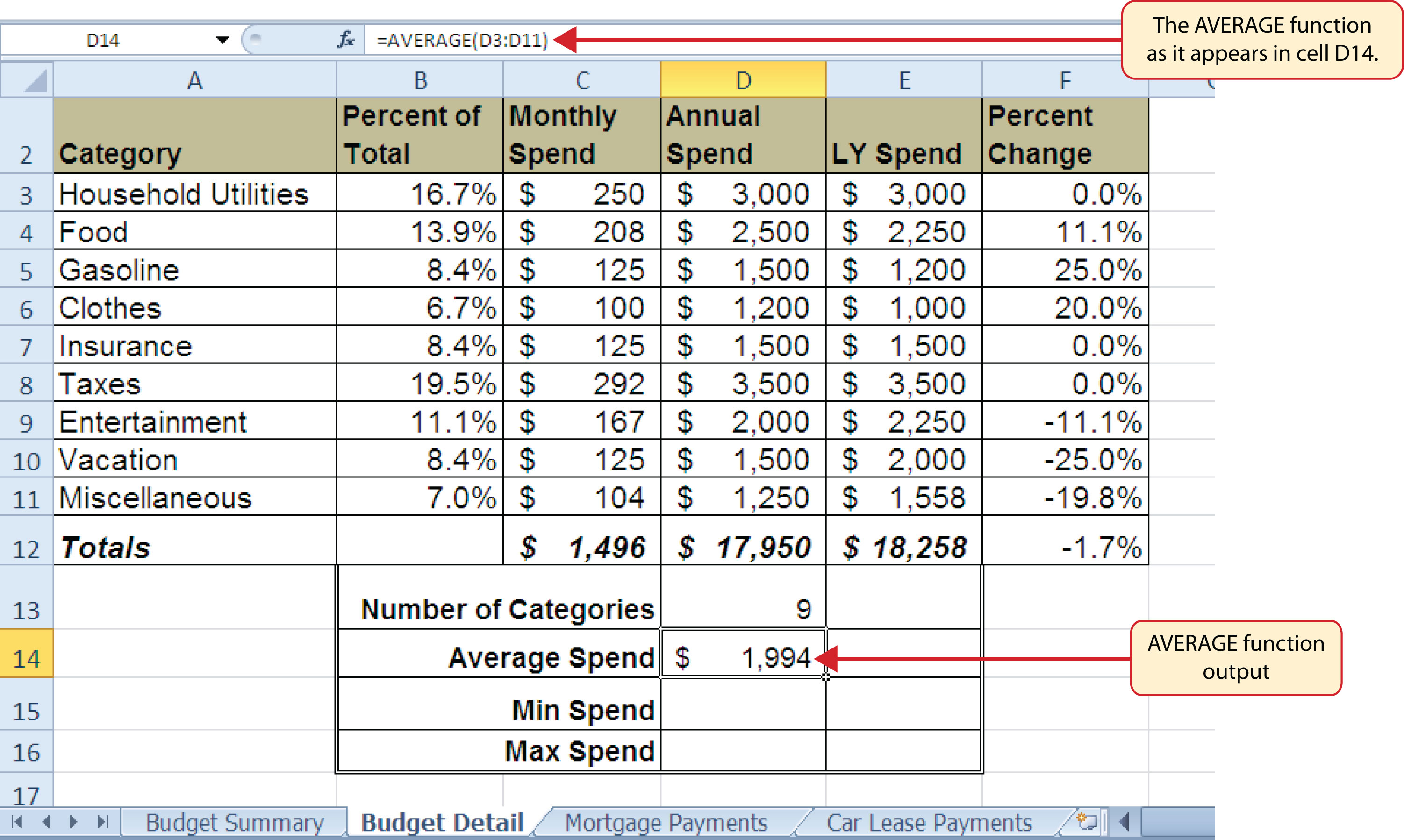## The MAX and MIN Functions

Follow-along file: Continue with Excel Objective 2.00. (Use file Excel Objective 2.07 if starting here.)

The final two statistical functions that we will add to the Budget Detail worksheet are the MAX and MIN functions. These functions identify the highest and lowest values in a range of cells. The following steps explain how to add these functions to the Budget Detail worksheet:

1. Click cell D15 in the Budget Detail worksheet.
2. Type an equal sign (=).
3. Type the word MIN.
4. Type an open parenthesis (().
5. Highlight the range D3:D11.
6. Type a closing parenthesis ()).
7. Press the ENTER key. The MIN function produces an output of \$1,200, which is the lowest value in the Annual Spend column (see Figure 2.24 "MIN Function Added to the Budget Detail Worksheet").
8. Click cell D16.
9. Type an equal sign (=).
10. Type the word MAX.
11. Type an open parenthesis (().
12. Highlight the range D3:D11.
13. Type a closing parenthesis ()).
14. Press the ENTER key. The MAX function produces an output of \$3,500. This is the highest value in the Annual Spend column (see Figure 2.25 "MAX Function Added to the Budget Detail Worksheet").

Figure 2.24 MIN Function Added to the Budget Detail Worksheet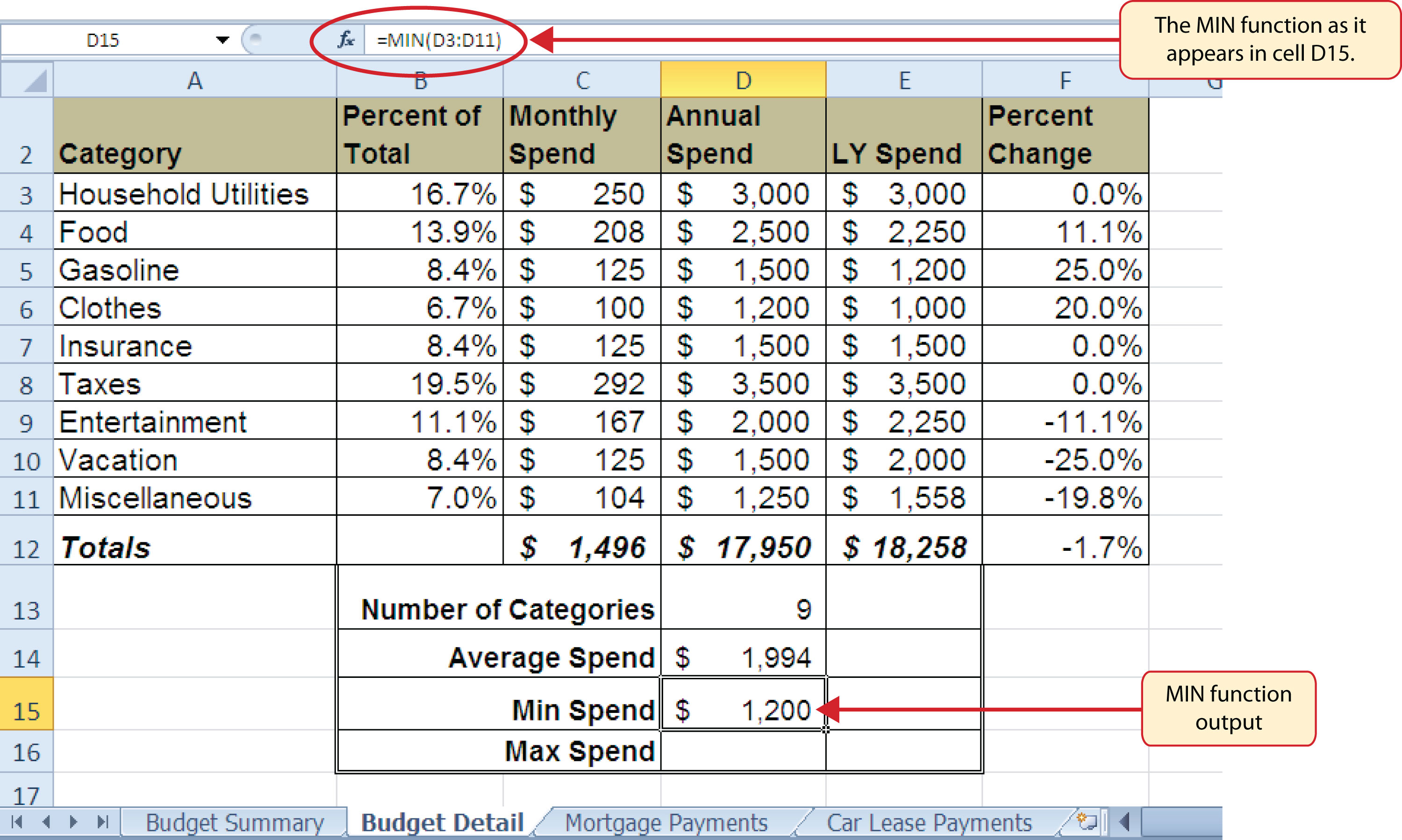Figure 2.25 MAX Function Added to the Budget Detail Worksheet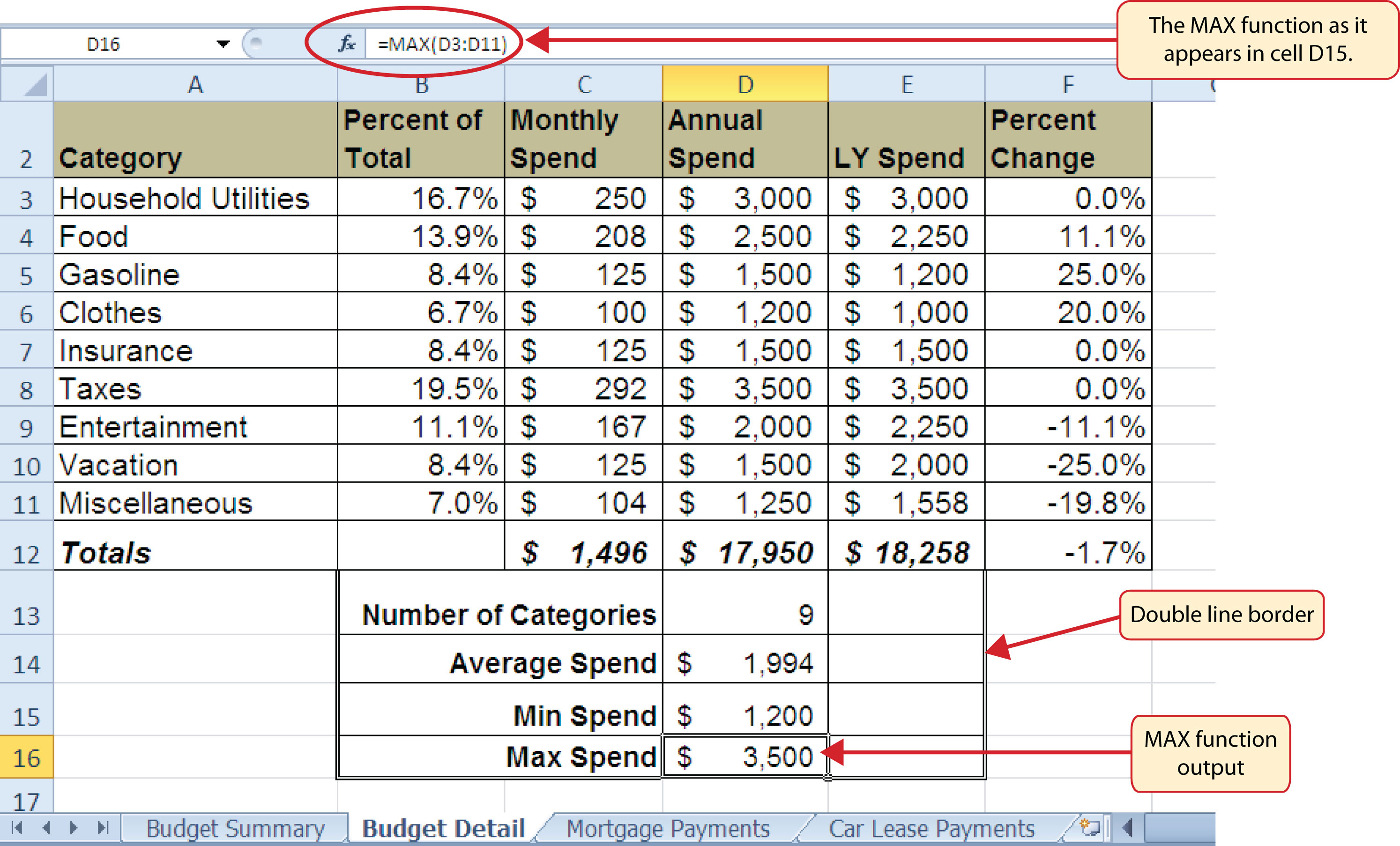### Skill Refresher: Statistical Functions

1. Type an equal sign (=).
2. Type the function name followed by an open parenthesis (() or double click the function name from the function list.
3. Highlight a range on a worksheet or click individual cell locations followed by commas.
4. Type a closing parenthesis ()).
5. Press the ENTER key.

## Copy and Paste Formulas (Pasting without Formats)

Follow-along file: Continue with Excel Objective 2.00. (Use file Excel Objective 2.08 if starting here.)

As shown in Figure 2.25 "MAX Function Added to the Budget Detail Worksheet", the COUNT, AVERAGE, MIN, and MAX functions are summarizing the data in the Annual Spend column. You will also notice that there is space to copy and paste these functions under the LY Spend column. This allows us to compare what we spent last year and what we are planning to spend this year. Normally, we would simply copy and paste these functions into the range E13:E16. However, you may have noticed the double-line style border that was used around the perimeter of the range B13:E16. If we used the regular Paste command, the double line on the right side of the range E13:E16 would be replaced with a single line. Therefore, we are going to use one of the Paste Special commands to paste only the functions without any of the formatting treatments. This is accomplished through the following steps:

1. Highlight the range D13:D16 in the Budget Detail worksheet.
2. Click the Copy button in the Home tab of the Ribbon.
3. Click cell E13.
4. Click the down arrow below the Paste button in the Home tab of the Ribbon.
5. Click the Formulas option from the drop-down list of buttons (see Figure 2.26 "Paste Formulas Option").

Figure 2.26 "Paste Formulas Option" shows the list of buttons that appear when you click the down arrow below the Paste button in the Home tab of the Ribbon. One thing to note about these options is that you can preview them before you make a selection by dragging the mouse pointer over the options. As shown in the figure, when the mouse pointer is placed over the Formulas button, you can see how the functions will appear before making a selection. Notice that the double-line border does not change when this option is previewed. That is why this selection is made instead of the regular Paste option.

Figure 2.26 Paste Formulas Option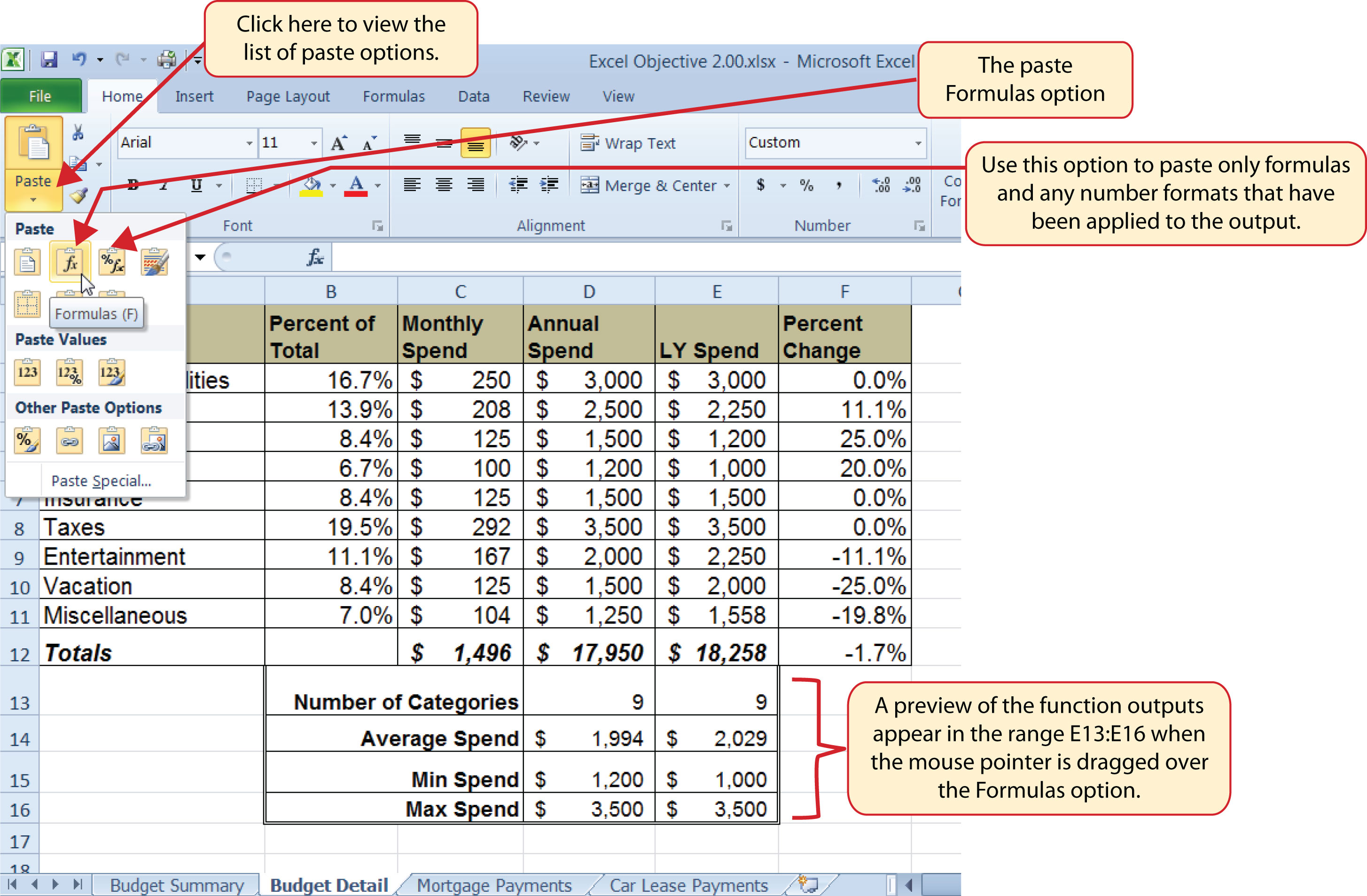### Skill Refresher: Paste Formulas

1. Click a cell location containing a formula or function.
2. Click the Copy button in the Home tab of the Ribbon.
3. Click the cell location or cell range where the formula or function will be pasted.
4. Click the down arrow below the Paste button in the Home tab of the Ribbon.
5. Click the Formulas button under the Paste group of buttons.

## Sorting Data (Multiple Levels)

Follow-along file: Continue with Excel Objective 2.00. (Use file Excel Objective 2.09 if starting here.)

The Budget Detail worksheet shown in Figure 2.26 "Paste Formulas Option" is now producing several mathematical outputs through formulas and functions. The outputs allow you to analyze the details and identify trends as to how money is being budgeted and spent. Before we draw some conclusions from this worksheet, we will sort the data based on the Percent of Total column. As mentioned in Chapter 1 "Fundamental Skills", sorting is a powerful tool that enables you to analyze key trends in any data set. We demonstrated the process of executing a single-level sort in Chapter 1 "Fundamental Skills". For the purposes of the Budget Detail worksheet, we need to set multiple levels for the sort order. This is accomplished through the following steps:

1. Highlight the range A2:F11 in the Budget Detail worksheet.
2. Click the Data tab in the Ribbon.
3. Click the Sort button in the Sort & Filter group of commands. This opens the Sort dialog box, as shown in Figure 2.27 "Sort Dialog Box".

Figure 2.27 Sort Dialog Box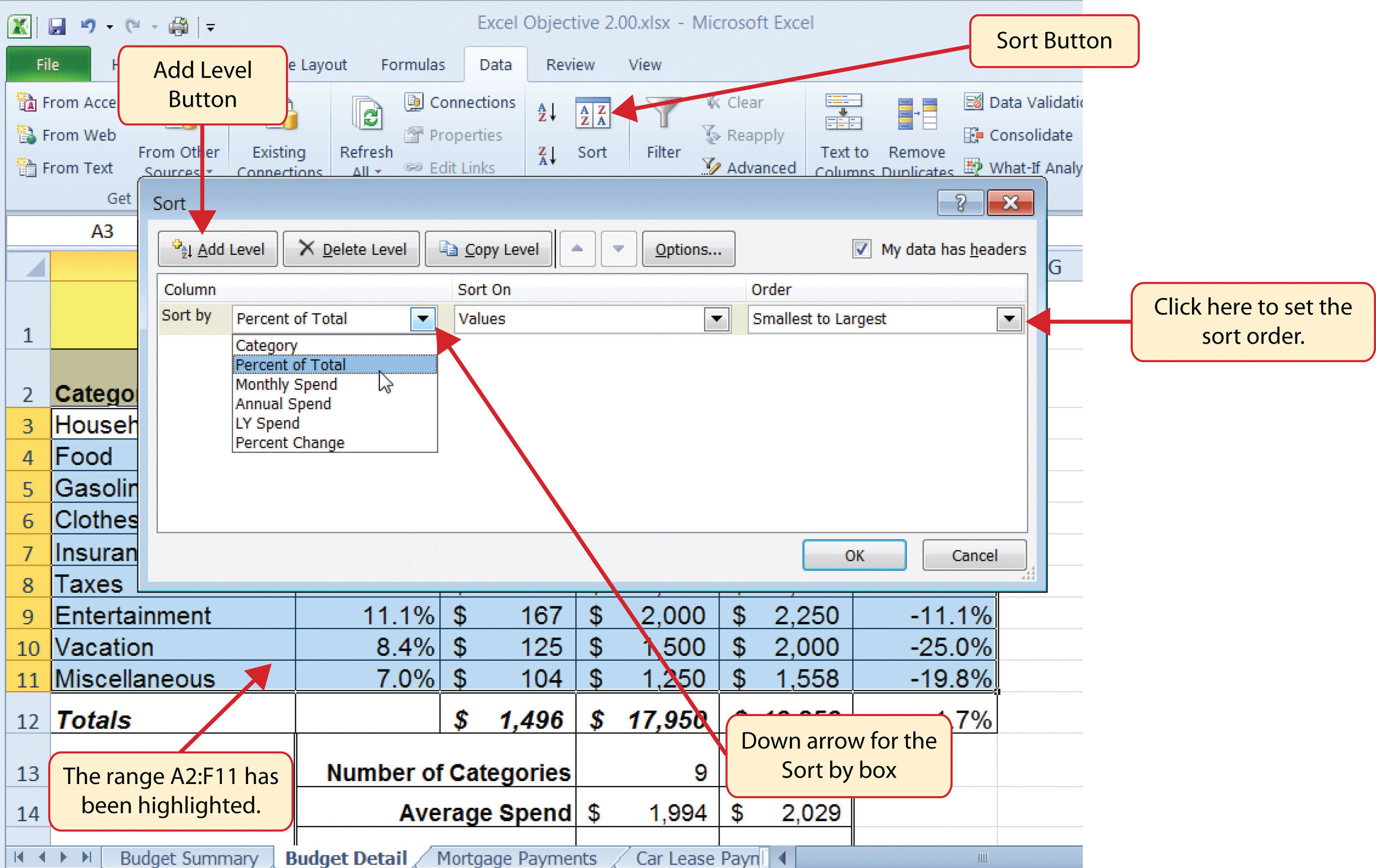4. Click the down arrow next to the “Sort by” box.
5. Click the Percent of Total option from the drop-down list.
6. Click the down arrow next to the sort Order box.
7. Click the Largest to Smallest option.
8. Click the Add Level button. This allows you to set a second level for any duplicate values in the Percent of Total column.
9. Click the down arrow next to the “Then by” box.
10. Select the LY Spend option.
11. Click the OK button at the bottom of the Sort dialog box.

Figure 2.28 "Budget Detail Worksheet after Sorting" shows the Budget Detail worksheet after it has been sorted. Notice that there are three identical values in the Percent of Total column. This is why a second sort level had to be created for this worksheet. The second sort level arranges the values of 8.4% based on the values in the LY Spend column in ascending order. Excel gives you the option to set as many sort levels as necessary for the data contained in a worksheet.

Figure 2.28 Budget Detail Worksheet after Sorting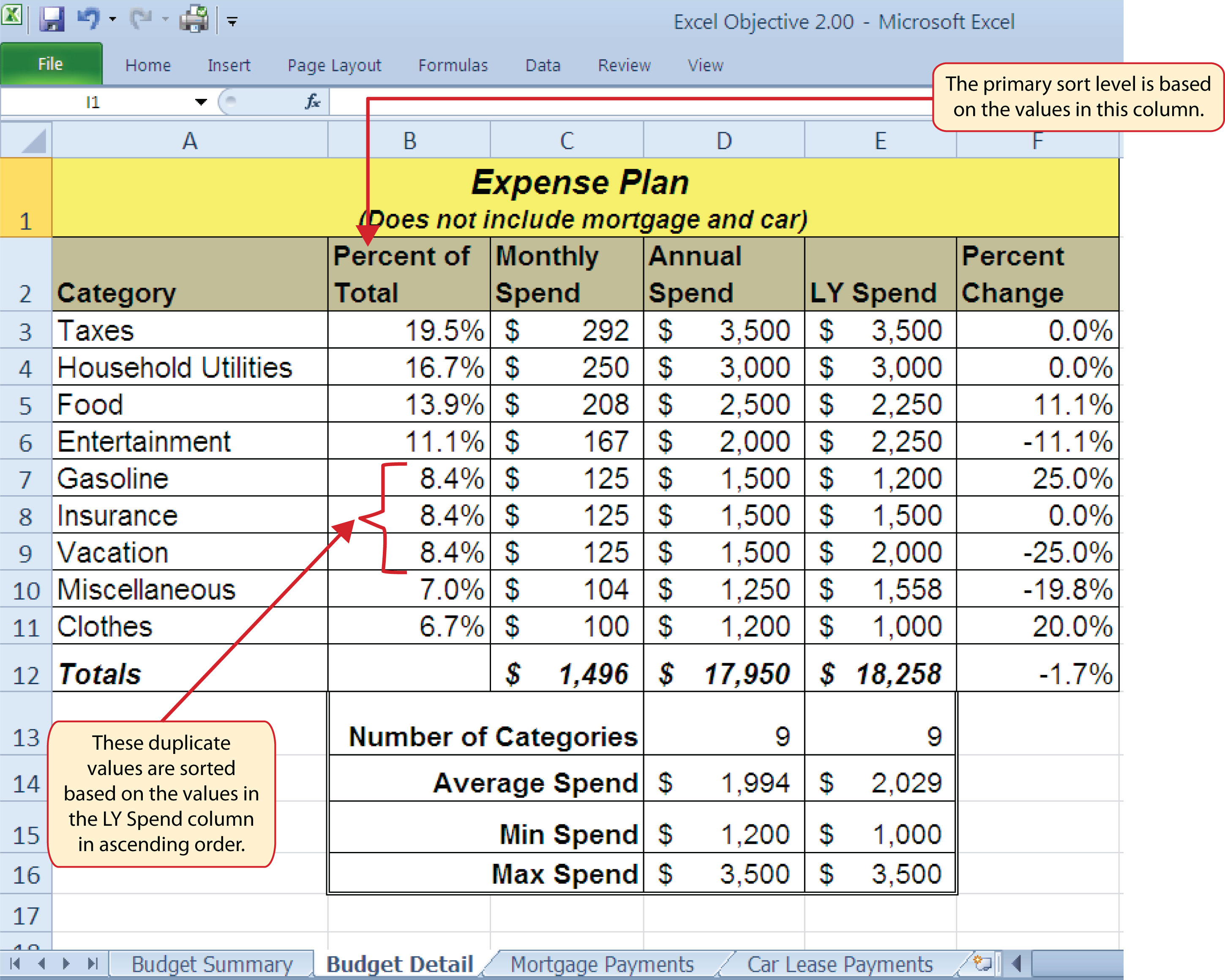### Skill Refresher: Sorting Data (Multiple Levels)

1. Highlight a range of cells to be sorted.
2. Click the Data tab of the Ribbon.
3. Click the Sort button in the Sort & Filter group.
4. Select a column from the “Sort by” drop-down list in the Sort dialog box.
5. Select a sort order from the Order drop-down list in the Sort dialog box.
6. Click the Add button in the Sort dialog box.
7. Repeat Steps 4 and 5.
8. Click the OK button on the Sort dialog box.

Now that the Budget Detail worksheet is sorted, a few key trends can be easily identified. The worksheet clearly shows that the top three categories as a percentage of total budgeted spending for the year are Taxes, Household Utilities, and Food. All three categories are necessities (or realities) of life and typically require a significant amount of income for most households. Looking at the Percent Change column, we can see how our planned spending is expected to change from last year. This is perhaps the most import column on the worksheet because it allows you to assess whether your plan is realistic. You will see that there are no changes planned for Taxes and Household Utilities. While Taxes can change from year to year, it is not too difficult to predict what they will be. In this case, we are assuming that there are no changes to the tax costs for our budget. We are also planning no change in Household Utilities. These costs can fluctuate from year to year as well. However, you can take measures to reduce costs, such as using less electricity, turning off heat when no one is in the house, keeping track of your wireless minutes so you do not go over the maximum allowed in your plan, and so on. As a result, there is no change in planned spending for Household Utilities because we will assume that any rate increases will be offset with a decrease in usage. The third item that is planned not to change is Insurance. Insurance policies for cars and homes can change, but as is true for taxes, the changes are predictable. Therefore, we are assuming no changes in our insurance policy.

The first big change that is noticeable in the worksheet is the Food and Entertainment categories in rows 5 and 6 (see definitions in Table 2.1 "Spend Category Definitions"). The Percent Change column indicates that there is an 11.1% decrease in Entertainment spending and an 11.1% increase in Food spending. This is logical because if you plan to eat in restaurants less frequently, you will be eating at home more frequently. Although this makes sense in theory, it may be hard to do in practice. Dinners and parties with friends may be tough to turn down. However, the entire process of maintaining a budget is based on discipline, and it certainly takes a significant amount of discipline to plan targets for yourself and stick to them.

A few other points to note are the changes in the Gasoline and Vacation categories. If you commute to school or work, the price of gas can have a significant impact on your budget. It is important to be realistic if gas prices are increasing, and you should reflect these increases in your budget. To compensate for the increased spending for gas, the spending plan for vacations has been reduced by 25%. Budgeting often requires a certain degree of creativity. Although the Vacation budget has been reduced, there is still money you can set aside to make plans for spring break or winter break.

Finally, the budget shows a decrease in Miscellaneous spending of 19.8%. This was defined as a group containing several expenses, such as textbooks, school supplies, software updates, and so on (see Table 2.1 "Spend Category Definitions"). You may be able to reduce your spending in this category if you can use items such as online textbooks. This reduction in spending can free up funds for Clothes, a spend category that has increased by 20%. We will continue to develop the Personal Budget workbook further in Section 2.3 "Functions for Personal Finance".

### Key Takeaways

• Statistical functions are used when a mathematical process is required for a range of cells, such as summing the values in several cell locations. For these computations, functions are preferable to formulas because adding many cell locations one at a time to a formula can be very time-consuming.
• Statistical functions can be created using cell ranges or selected cell locations separated by commas. Make sure you use a cell range (two cell locations separated by a colon) when applying a statistical function to a contiguous range of cells.
• To prevent Excel from changing the cell references in a formula or function when they are pasted to a new cell location, you must use an absolute reference. You can do this by placing a dollar sign (\$) in front of the column letter and row number of a cell reference.
• The #DIV/0 error appears if you create a formula that attempts to divide a constant or the value in a cell reference by zero.
• The Paste Formulas option is used when you need to paste formulas without any formatting treatments into cell locations that have already been formatted.
• You need to set multiple levels, or columns, in the Sort dialog box when sorting data that contains several duplicate values.

### Exercises

1. In the formula =C2/\$C\$24, the dollar signs used in the C24 cell reference indicate:

1. Relative referencing has been turned off for this cell reference.
2. Any value entered into cell C24 will be formatted with US currency.
3. The output of the formula will be formatted with US currency.
4. The value currently in C24 cannot be changed.
2. Which statement best explains how the following function will produce a result =AVERAGE(B1,B10)?

1. The function will calculate the average for the values in B1 and B10.
2. The function will calculate the average for the values in all cell locations in the range B1 through and including B10.
3. Commas cannot be used in statistical functions. The function cannot produce an output and will display the #DIV/0 error code.
4. The function will take the average of cell B1 based on the value that is entered into cell B10.
3. Which of the following best explains the purpose of the Paste Formulas command?

1. You cannot use the regular Paste command for formulas and functions. You need to use the Paste Formulas command when you copy and paste a formula or function to additional cell locations on a worksheet.
2. You would use the Paste Formulas command when you want to show the formula or function in a cell location and not the calculated output.
3. You would use the Paste Formulas command when you want to paste only the formula or function to a new cell location without the formatting treatments that were applied to the copied cell location.
4. The Paste Formulas command is required if you want to paste a formula into multiple cell locations on a worksheet.
4. Which of the following explains how data in a worksheet can be sorted if the primary column of data contains several duplicate values?

1. You can only sort data based on the values in one column. Excel will take the duplicate values and sort them in the order in which they were entered into the worksheet.
2. Excel will sort any duplicate values in the primary column based on the values one column to the right in ascending order.
3. You can click the Add button in the Sort dialog box and designate which column Excel should use to sort any duplicate values in the primary column.
4. You would sort each column in the worksheet one at a time.